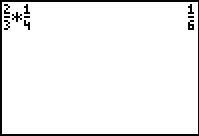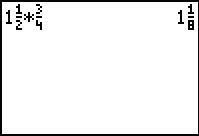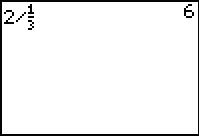# Activities

••• ##### Subject Area

• Math: Middle Grades Math: Number Sense

• ##### Author6-8

45 Minutes

• ##### Device
• TI-73 Explorer™
• TI-Navigator™
• ##### Software

TI Connect™
TI-SmartView™

• ##### Accessories

TI Connectivity Cable

• ##### Other Materials
Rational Number Rampage

## Fractions with Visual Models#### Activity Overview

In this activity, students will explore multiplication and division of fractions using several representations to develop fluency. Students will also solve problems involving similarity using proportions.

#### Key Steps

•Students will enter fractions and multiply. To do this, they need to follow the directions on the worksheet, entering the numerator, the fraction key, the denominator, the multiplication operation, then the second fraction.

•To enter a mixed number, student will use the "Unit" key.

•Many students have difficulty understanding what is being done when dividing by a fraction. It can be explained as finding how many “divisors” are in the “dividend”. Using the fraction tiles and TI-73 Explorer should help students visualize this concept.